# orthfilt

Orthogonal wavelet filters

## Syntax

``[Lo_D,Hi_D,Lo_R,Hi_R] = orthfilt(W)``

## Description

example

````[Lo_D,Hi_D,Lo_R,Hi_R] = orthfilt(W)` computes the four filters associated with the scaling filter `W` corresponding to a wavelet. The four filters the function computes are decomposition lowpass filter `Lo_D`, decomposition highpass filter `Hi_D`, reconstruction lowpass filter `Lo_R`, and reconstruction highpass filter `Hi_R`.For more information on how the function computes the filters, see Algorithms.```

## Examples

collapse all

Create a scaling filter associated with the Daubechies wavelet specified by `'db8'`.

```W = dbwavf('db8'); stem(W); title('Original scaling filter');```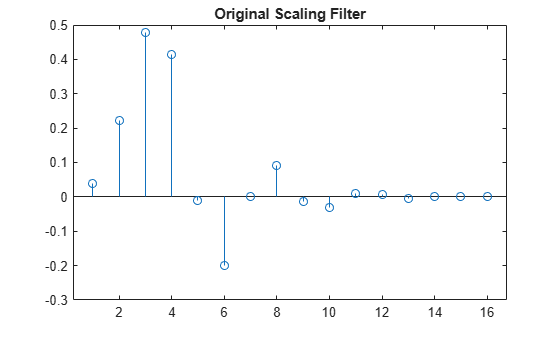Compute the four filters associated with the scaling filter.

`[Lo_D,Hi_D,Lo_R,Hi_R] = orthfilt(W); `

Plot the decomposition lowpass filter.

```stem(Lo_D); title('Decomposition lowpass filter'); ```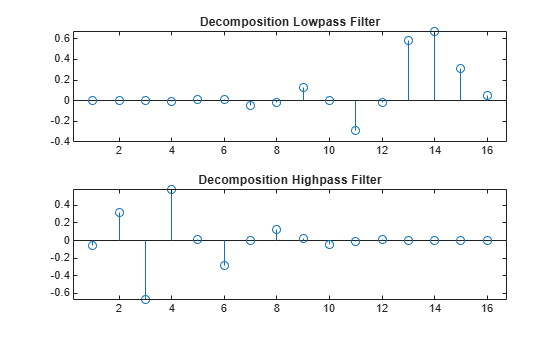Plot the decomposition highpass filter.

```stem(Hi_D); title('Decomposition highpass filter'); ```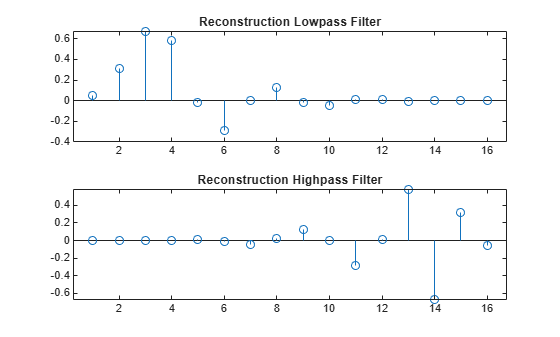Plot the reconstruction lowpass filter.

```stem(Lo_R); title('Reconstruction lowpass filter'); ```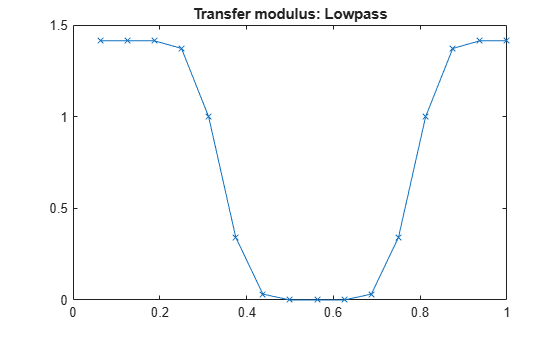Plot the reconstruction highpass filter.

```stem(Hi_R); title('Reconstruction highpass filter');```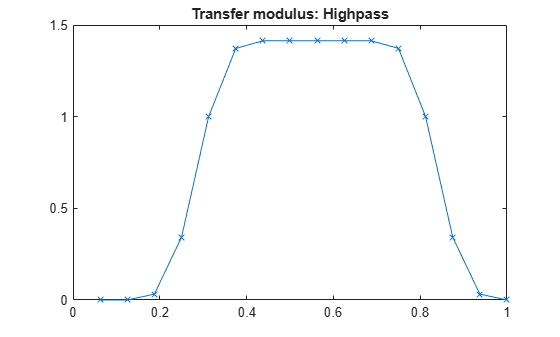Check for orthonormality in the decomposition filters..

```df = [Lo_D;Hi_D]; rf = [Lo_R;Hi_R]; id = df*df'```
```id = 2×2 1.0000 -0.0000 -0.0000 1.0000 ```

Check for orthonormality in the reconstruction filters..

`id2 = rf*rf'`
```id2 = 2×2 1.0000 0.0000 0.0000 1.0000 ```

Check for orthogonality by dyadic translation.

```df = [Lo_D 0 0;Hi_D 0 0]; dft = [0 0 Lo_D; 0 0 Hi_D]; zer = df*dft'```
```zer = 2×2 10-12 × -0.1895 0.0000 0.0000 -0.1895 ```

Plot the low-frequency transfer modulus.

```fftld = fft(Lo_D); freq = [1:length(Lo_D)]/length(Lo_D); plot(freq,abs(fftld)); title('Transfer modulus: low-pass'); ```Plot the high-frequency transfer modulus.

```ffthd = fft(Hi_D); plot(freq,abs(ffthd)); title('Transfer modulus: high-pass')```## Input Arguments

collapse all

Scaling filter corresponding to a wavelet, specified as a real-valued vector.

## Output Arguments

collapse all

Decomposition lowpass filter associated with the scaling filter `W`, returned as a real-valued vector.

Decomposition highpass filter associated with the scaling filter `W`, returned as a real-valued vector.

Reconstruction lowpass filter associated with the scaling filter `W`, returned as a real-valued vector.

Reconstruction highpass filter associated with the scaling filter `W`, returned as a real-valued vector.

## Algorithms

For an orthogonal wavelet in the multiresolution framework, start with the scaling function ϕ and the wavelet function ψ. One of the fundamental relations is the twin-scale relation:

`$\frac{1}{2}\varphi \left(\frac{x}{2}\right)=\sum _{n\in Z}{w}_{n}\varphi \left(x-n\right)$`

All the filters used in the `dwt` and `idwt`functions are intimately related to the sequence ${\left({w}_{n}\right)}_{n\in Z}$. if ϕ is compactly supported, the sequence (wn) is finite and can be viewed as an FIR filter. The scaling filter `W` is a lowpass FIR filter of length 2N, with the sum 1, and with the norm of 1/√2."

For example, for a `db3` scaling filter,

```w = dbwavf('db3') w = 0.2352 0.5706 0.3252 -0.0955 -0.0604 0.0249 sum(w) = 1.000 norm(w) = 0.7071 ```

Define four FIR filters from filter `W` of length 2N and norm 1.

Filters

Low-Pass

High-Pass

Decomposition

`Lo_D``Hi_D`

Reconstruction

`Lo_R``Hi_R`

The function computes the four filters using the following scheme.The algorithm defines `qmf` is such that `Hi_R` and `Lo_R` are quadrature mirror filters (that is ```Hi_R(k) = (-1)k````Lo_R(2N + 1 - k)`, for `k = 1, 2, Ä, 2N`) and `wrev` such that it flips the filter coefficients. Therefore, `Hi_D` and `Lo_D` are also quadrature mirror filters.

 Daubechies, I. (1992). Ten lectures on wavelets. CBMS-NSF conference series in applied mathematics, SIAM Ed. pp. 117–119, 137, 152.

## SupportGet trial now